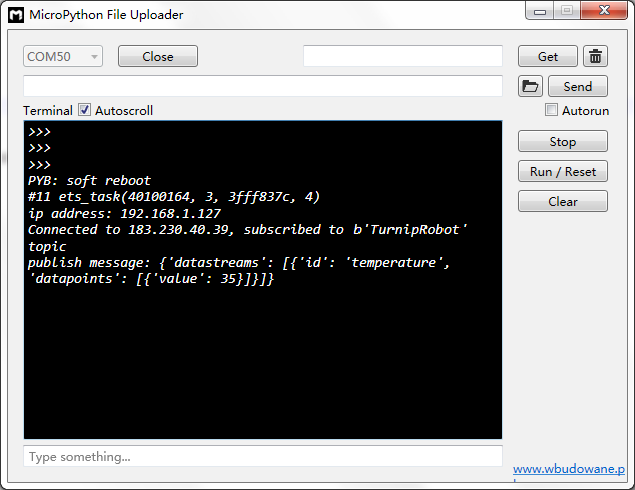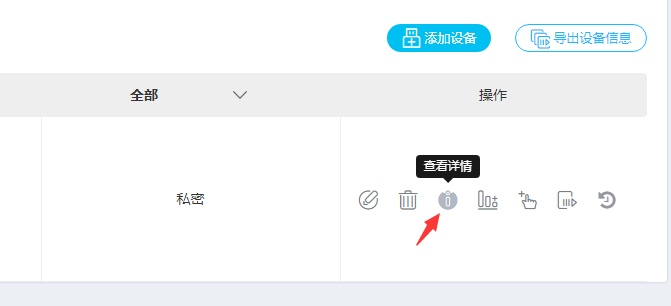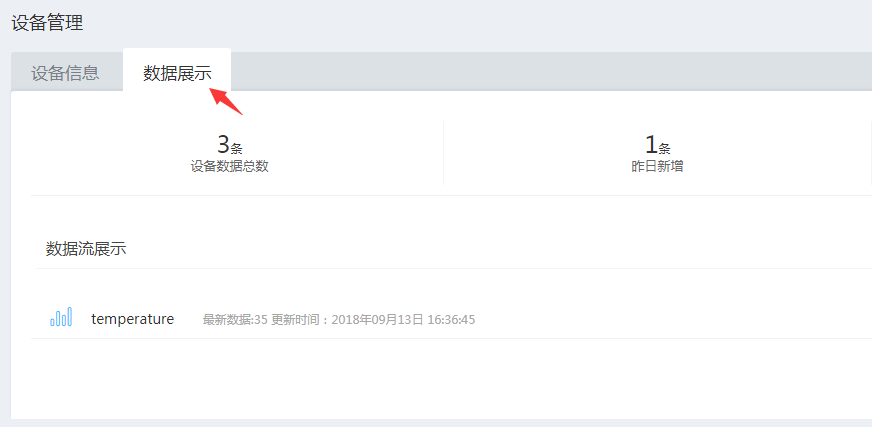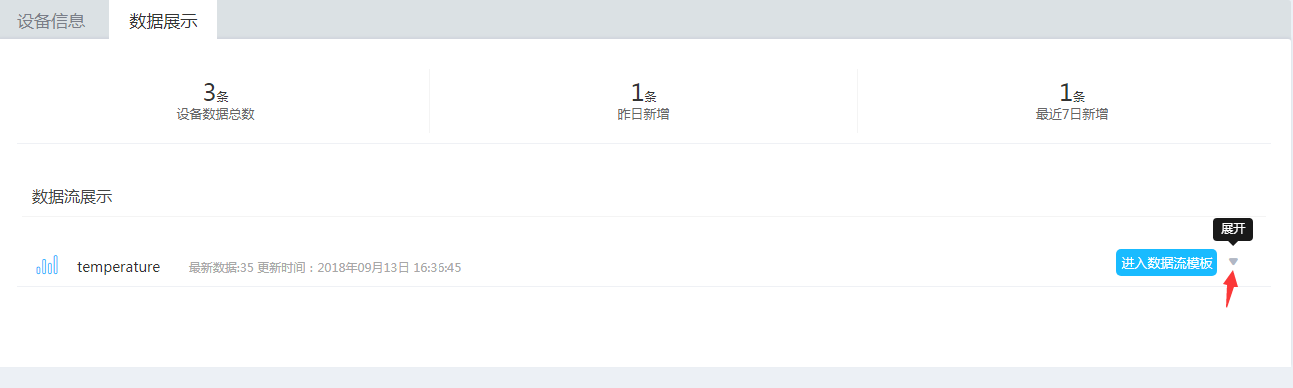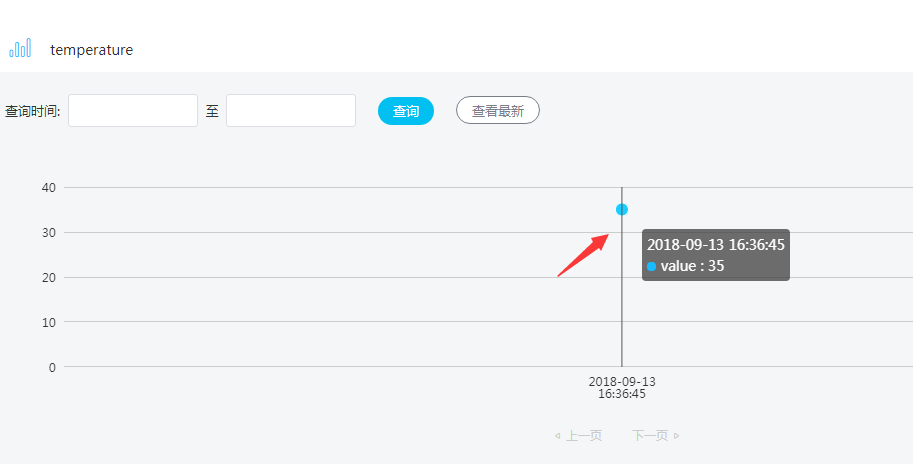# 6. [Micropython]TPYBoard v202 MQTT协议2:上传数据点到OneNET平台¶

## 6.2. 准备工作¶

• TPYBoard v202开发板
• USB数据线

## 6.3. OneNET平台新建数据流¶

OneNET平台官网地址：https://open.iot.10086.cn/。 若没有使用过的该平台的，可以参考上面前言中的教程，先进行产品和设备的添加。

1、进入[开发者中心]的[设备管理]页面，在左侧菜单栏中选择[数据流模板]，点击[添加数据流]。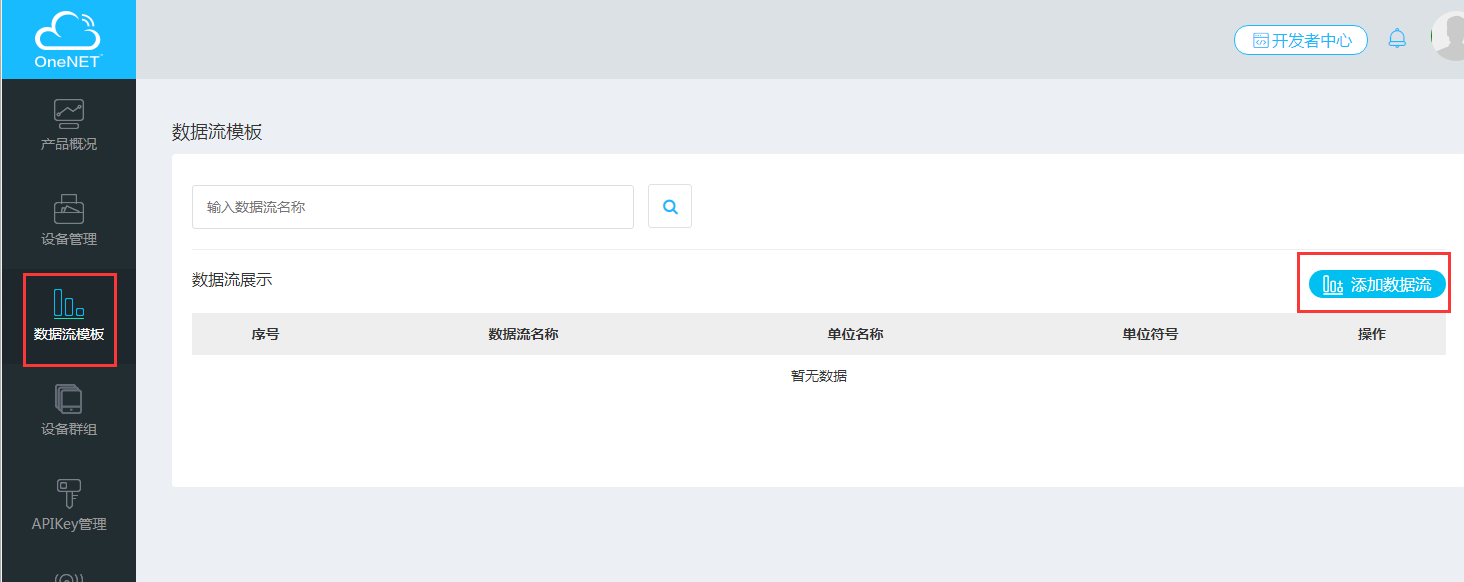2、会弹出一个添加数据流的对话框，必填项名称，其他可以选填，填完之后点击[添加]。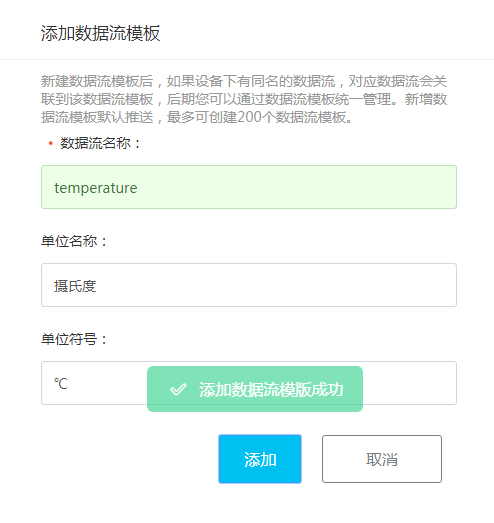3、添加成功后，对话框会自动关闭。数据流展示列表会自动增加刚才新添加的数据流信息。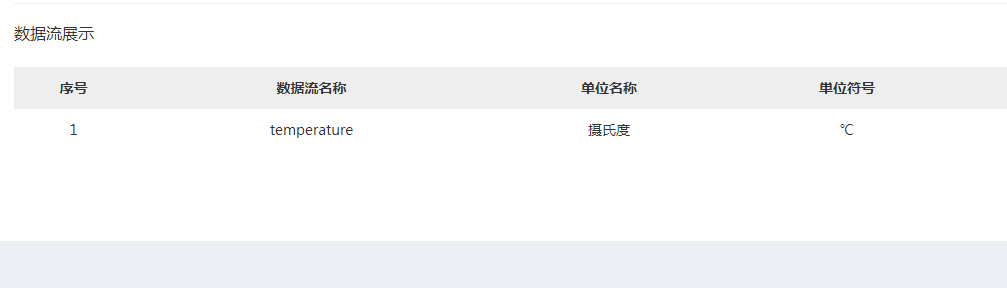## 6.4. 向OneNET平台上传数据点¶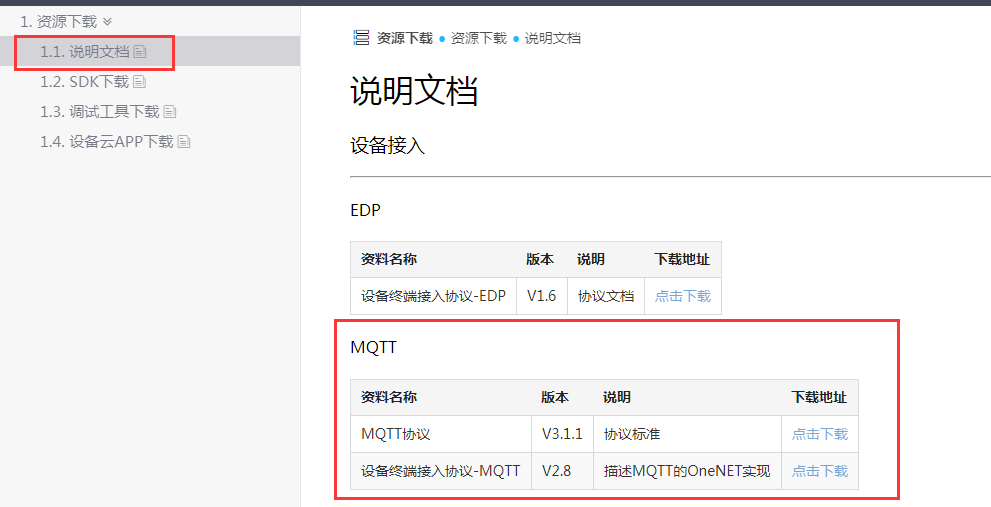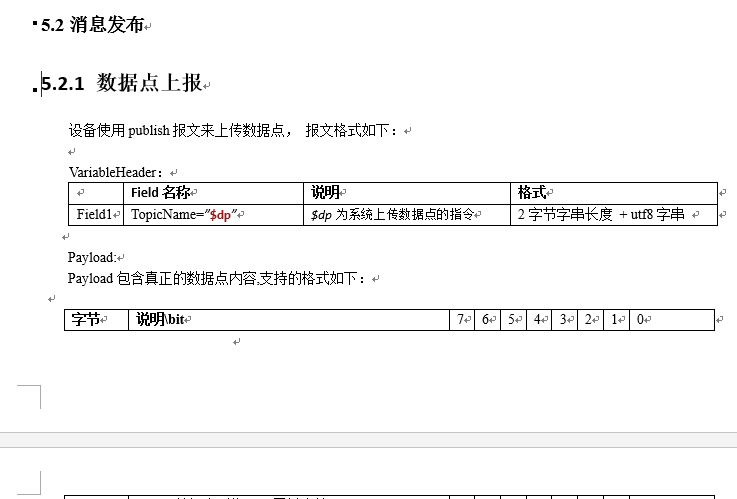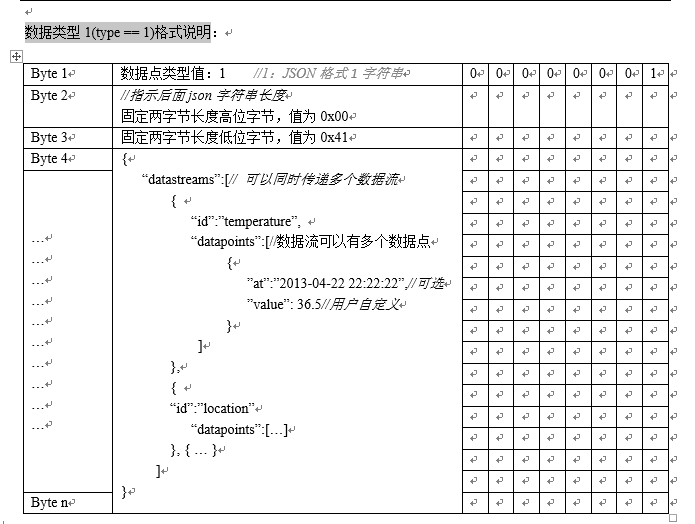```from simple import MQTTClient
from machine import Pin
import machine
import micropython
import json

#选择G4引脚
g4 = Pin(4, Pin.OUT, value=0)
# MQTT服务器地址域名为：183.230.40.39,不变
SERVER = "183.230.40.39"
#设备ID
CLIENT_ID = "deviceID"
#随便起个名字
TOPIC = b"TurnipRobot"
#产品ID
#产品APIKey:
state = 0
#要上报的数据点
message = {'datastreams':[{
'id':'temperature',
'datapoints':[{'value':35}]}
]}

def pubdata(data):
j_d = json.dumps(data)
j_l = len(j_d)
arr = bytearray(j_l + 3)
arr = 1 #publish数据类型为json
arr = int(j_l / 256) # json数据长度 高位字节
arr = j_l % 256      # json数据长度 低位字节
arr[3:] = j_d.encode('ascii') # json数据
return arr

def sub_cb(topic, msg):
global state
print((topic, msg))
if msg == b"on":
g4.value(1)
state = 1
print("1")
elif msg == b"off":
g4.value(0)
state = 0
print("0")
elif msg == b"toggle":
state = 1 - state
g4.value(state)

def main(server=SERVER):
#端口号为：6002
c.set_callback(sub_cb)
c.connect()
c.subscribe(TOPIC)
print("Connected to %s, subscribed to %s topic" % (server, TOPIC))
#publish报文上传数据点
c.publish('\$dp',pubdata(message))
print('publish message:',message)

try:
while 1:
c.wait_msg()
finally:
c.disconnect()
```

```def pubdata(data):
j_d = json.dumps(data)
j_l = len(j_d)
arr = bytearray(j_l + 3)
arr = 1 #publish数据类型为json
arr = int(j_l / 256) # json数据长度 高位字节
arr = j_l % 256      # json数据长度 低位字节
arr[3:] = j_d.encode('ascii') # json数据
return arr
```

```c.publish('\$dp',pubdata(message))
```

## 6.5. 效果展示¶